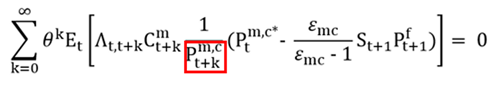# Loss function and OSR command

Hi everyone,
I am currently working on a model similar to ALLV (2005), but more simple, from what i understand, in the Ramses model, the steady state is inneficient so, could it be posible to use the ramsey_model or the osr commands? or i need and efficient steady state? My plan is to estimate and evaluate different monetary policy rules, but i am stuck with that part.

The issue with the inefficient steady state is mostly about the approximation order required. For `ramsey_model` that is not an issue. You can simply go to second order. The `osr`-command in contrast only works with linear models, so a inefficient steady state is a problem. But you could implement OSR manually at higher order.

I followed your approach and changed my model to a non-linear form, I learned how to recursively derive calvo price equation, however i have a small doubt, in one of your examples, the price FOC is as follows:and for the importing firms in an incomplete pass-through environment, following your notation, the FOC of the importing companies would be:or do I need to divide by the general price level instead of the general price of imported goods?

Thanks!

The deflator is put here to be able to use the real stochastic discount factor \Lambda_{t,t+k}. That factor applies to real cash flows in terms of the final good. Thus, the deflator needs to be the one of the consumption good.

That makes sense, thanks a lot professor.

Best regards

Hi all

I am working with a similar model (Adolfson, et al. 2005), so I would like to know if another solution to this kind of problem would be to add non-distortionary taxes to restore the efficient steady state to be able to use the osr command? If so, in the case of models like this one, where there is monopolistic competition in several sectors (domestic sector, import and export sector, etc), to restore the efficient steady state, is it valid to just add a lump sum tax to each sector?